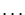Next: Interrupting Computation Up: Exploring the Basics Previous: The If Command

# Looping Around

For a programming language to be of any use, it must include some ability to loop through a set of statements. Recall that a statement in Darwin is a command or expression followed by a terminating symbol (the semicolon or colon). A command sequence is a sequence of commands separated by terminating symbols. The terminating symbol after the last command in a command sequence is optional. The for command is our first example of iteration in Darwin.

```> X:=CreateArray(1..100):
> for i from 1 to length(X) do
>   X[i]:=i:                                     # a command sequence
>   lprint('The value of X[', i, '] is ', X[i])  # no terminating semicolon
>                                                #   or colon needed
> od:
The value of X[ 1 ] is  1
The value of X[ 2 ] is  2
The value of X[ 3 ] is  3
The value of X[ 4 ] is  4
.
.
.
```
After the for loop terminates, the ith element of X contains the number i. Of course, we could have accomplished the same by writing one hundred different statements of the form X:=1, X:=2,, X:=100. The body of the loop consists of the command sequence between the dood commands.

Now suppose we only want to print those elements of X which are odd and divisible by both 3 and 5. Since all elements of X which have an even index are assigned an even integer, we need only check the odd elements of X to see if they are divisible by these numbers.

```> for i from 1 to length(X) by 2 do
>   if ((mod(X[i], 3)=0) and (mod(X[i], 5)=0)) then
>     lprint('X[',i,']=',X[i],' is divisible by both 3 and 5');
>   fi;
> od;
X[ 15 ]= 15  is divisible by both 3 and 5
X[ 45 ]= 45  is divisible by both 3 and 5
X[ 75 ]= 75  is divisible by both 3 and 5
```
Here mod(x,y) denotes the modulus function; this returns the integer remainder after dividing y into x. Note the use of the by 2 clause in the for command. In general, you may count in steps by any value you would like. We may also specify a negative increment to count backwards. If we change the first line of the about for loop to
```> for i from length(X)-1 to 1 by -2 do
```
then the exact same is accomplished but in the reverse order.
```X[ 75 ]= 75  is divisible by both 3 and 5
X[ 45 ]= 45  is divisible by both 3 and 5
X[ 15 ]= 15  is divisible by both 3 and 5
```

Chapterdescribes several other means of looping in Darwin.Next: Interrupting Computation Up: Exploring the Basics Previous: The If Command
Gaston Gonnet
1998-09-15